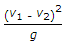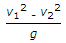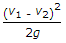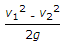# Mechanical Engineering - Hydraulics and Fluid Mechanics

### Exercise :: Hydraulics and Fluid Mechanics - Section 1

11.

The length AB of a pipe ABC in which the liquid is flowing has diameter (d1) and is suddenly enlarged to diameter (d2) at B which is constant for the length BC. The loss of head due to sudden enlargement is

 A.B.C.D.Explanation:

No answer description available for this question. Let us discuss.

12.

Coefficient of contraction is the ratio of

 A. actual velocity of jet at vena contracta to the theoretical velocity B. loss of head in the orifice to the head of water available at the exit of the orifice C. actual discharge through an orifice to the theoretical discharge D. area of jet at vena contracta to the area of orifice

Explanation:

No answer description available for this question. Let us discuss.

13.

In a free nappe,

 A. the pressure below the nappe is atmospheric B. the pressure below the nappe is negative C. the pressure above the nappe is atmospheric D. the pressure above the nappe is negative

Explanation:

No answer description available for this question. Let us discuss.

14.

The Reynold's number of a ship is __________ to its velocity and length.

 A. directly proportional B. inversely proportional

Explanation:

No answer description available for this question. Let us discuss.

15.

When a tube of smaller diameter is dipped in water, the water rises in the tube due to viscosity of water.

 A. True B. False